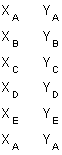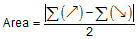## 2. Area By Coordinates

### a. Concept

The area of a closed non-crossing plane polygon can be compuetd from the coordinates of the polygon's verticies.The area equations, depending on coordinate format used, are shown here as Equations G-1 and G-2

 In terms of X and Y coordinatesEquation G-1 In terms of N and E coordinatesEquation G-2

These equations look complex, more so if you expand all the terms. Rather than memorize each equation, it's easier to remember their pattern and determine the area in tabular fashion.

To accurately compte the traverse area:

(1) Select a start point (it doesn't matter where you start)

(2) Going in sequence around the exterior list each coordinate pair vertically, Figure G-5. Direction of travel around the traverse (clockwise or counter-clockwise) doesn't matter; nor does coordinate precedence, eg, (X, Y) or (Y, X).

 Using X,Y coords Using N,E coordsFigure G-5 LIsting Coordinates in Sequence

(3) The first coordinate pair must be repeated at the bottom of the list. In Figure G-5, the surveyor started at point A and then ended on point A.

(4) Cross multiply the coordinates and sum the products, Figure G-6. Arrows indicate "direction" of multiplication.

 Using X,Y coords Using N,E coordsFigure G-6 Coordinate Crossmultiplication

The units of the cross products are square linear units - if coordinates are in feet, cross products are sq ft.

(5) Using Equation G-3, compute the traverse area.

Sum each column () and ()Equation G-3

The absolute value is used since the area could be negative depending on the combination of direction around the traverse, coordinate precedence (e.g. X,Y vs Y,X), and cross multiplication order. Just as the square root of 4 can be either +2 or -2, so can the area be positive or negative. Because we're generally interested in the magnitude of the answer, we use the absolute value of the area computed..

While at first all this may look confusing, it's actually pretty easy to remember once you do it a few times.

### b. Examples

Although we carried an additional digit in all previous computations, we'll carry a few more extra here. We'll discuss the area error at the end of the chapter but for now we want to over-compute the area accuracy then report it to an appropriate resolution after we analyze it. If we don't carry additional digits, we could easily increase error due to rounding. To be on the safe side, we'll carry the computations to 0.1 which should be less than the expected error.

#### (1) Example 1

Figure G-7 is a continuation of the Bearing Traverse example we have been using in the past few chapters.Point North (ft) East (ft) A 500.000 2000.000 B 323.614 1561.426 C 526.996 1488.321 D 719.336 1686.956
Figure G-7
Example 1 Traverse

Step (1) Start at point A and going clockwise around the traverse list the coordinates:

 Point N (ft) E (ft) A 500.000 2000.000 B 323.614 1561.426 C 526.996 1488.321 D 719.336 1686.956 A 500.000 2000.000 remember to return to A

Step (2) Cross multiply in one direction:

 Point N (ft) E (ft) (), sq ft A 500.000 2000.000 647,228.0 B 323.614 1561.426 822,865.2 C 526.996 1488.321 1,070,602.9 D 719.336 1686.956 843,478.0 A 500.000 2000.000

Step (3) Cross multiply in the other direction

 Point N (ft) E (ft) (), sq ft (), sq ft A 500.000 2000.000 647,228.0 B 323.614 1561.426 822,865.2 780,713.0 C 526.996 1488.321 1,070,602.9 481,641.5 D 719.336 1686.956 843,478.0 889,019.1 A 500.000 2000.000 1,438,672.0

Step (4) Add up the columns

 Point N (ft) E (ft) (), sq ft (), sq ft A 500.000 2000.000 647,228.0 B 323.614 1561.426 822,865.2 780,713.0 C 526.996 1488.321 1,070,602.9 481,641.5 D 719.336 1686.956 843,478.0 889,019.1 A 500.000 2000.000 1,438,672.0 sums: 3,384,174.1 3,590,045.6

Step (5) Using Eqn (G-3) compute the areaUntil we discuss area accuracy more fully, we'll state the area as 102,935.8 sq ft.

There's nothing magical or sacred about point A. We could have stated our list at point C and travelled counter-clockwiase around the traverse. As long as we remember to repeat the intial point at the bottom of the list, we will come up with the same area although one could be positive and the other negative.

#### (2) Example 2

Figure G-8 shows the Crossing Traverse we've been using as another running example.Point North (ft) East (ft) E 1000.000 200.000 F 689.206 532.694 G 896.890 627.584 H 692.474 257.460
Figure G-8
Crossing Traverse

With a crossing traverse, one must be careful when listing the coordinates. In this case, if you list the coordinates along the original traverse path, E-F-G-H-E, you will be able to compute an area but it will be nonsensical. The traverse turns itself inside out.

Recall that this survey was on a four-sided parcel having two obstructed lines, Figure G-9.Figure G-9 Parcel With Obstructions

We want the the area of the parcel, not the traverse.

Step (1) Start at point E and going clockwise around the parcel list the coordinates.

 Point N (ft) E (ft) E 1000.000 200.000 G 896.890 627.584 F 689.206 532.694 H 692.474 257.460 E 1000.000 200.000 remember to return to E

Step (2)-(4) Cross multiply in both directions; sum the columns.

 Point N (ft) E (ft) (), sq ft (), sq ft E 1000.000 200.000 179,378.0 G 896.890 627.584 432,534.7 624,584.0 F 689.206 532.694 368,876.7 477,767.9 H 692.474 257.460 257,460.0 177,443.0 E 1000.000 200.000 138,494.8 1,238,252.4 1,418,289.7

Step (5) Use Equation (G-3) to compute the area